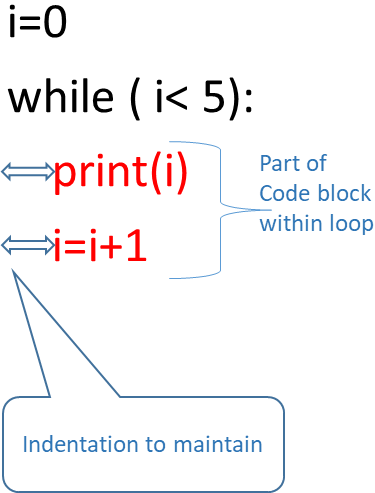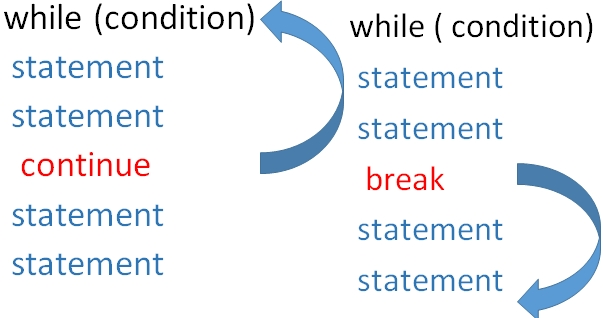# While Loop in Python

While loop
``````i=0
while ( i< 5):
print(i)
i=i+1``````Note that the 2nd line print(i) is placed after an indente of one space. Without that we will get an error asking expectd an indented block . The code to be executed within the block is to be kept with indenting.
The loop will continue as long as the condition is true. We used one incremental variable i to change the condition to match the loop. ( In For loop we have not used any incremental variable )

The output of above code is here
``````0
1
2
3
4``````

Python while loop with else break & continue to execute code blocks based on condition True or False

## While loop with else

Code wihin else will execute once the loop is over
``````i=0;
while ( i< 5):
print(i)
i=i+1
else:
print("i am outside the loop")``````
``````0
1
2
3
4
i am outside the loop``````

## Break and continue in a while loop

When break statement is encountered the execution comes out of the loop. In case of continue the execution returns to the starting of the loop skipping the rest of the statements ( after continue ) and continues again.

In the codes give below , as soon as break is encountered the execution comes out of the loop, so 3,4 and 5 are not printed. But when continue is used in place of break the printing of 3 is skipped but the loop continues again so 4 and 5 are printed.

You can also observe the else part of the code is not executed once break statement is encounterd and execution comes out of the loop.Using continue
``````i=0;
while ( i< 5):
i=i+1
if(i==3):
continue
print(i)
else:
print("i am outside the loop")``````
``````1
2
4
5
i am outside the loop``````
Using break
``````i=0;
while ( i< 5):
i=i+1
if(i==3):
break
print(i)
else:
print("i am outside the loop")``````
``````1
2``````

## Checking if input number is prime number or not

``````num=int(input("Enter the number you want to check : "))
i=2
while(i<num/2):
if(num%i == 0):
print(num, " is not a prime number")
break;
i=i+1
else:
print(num , " is a prime number")``````
In above code if the condition checked for if became True for any value of i then the break statement will be executed terminating the while loop and the else part of the code will be skipped.

Subscribe to our YouTube Channel here

## Subscribe

* indicates required
Subscribe to plus2netplus2net.com